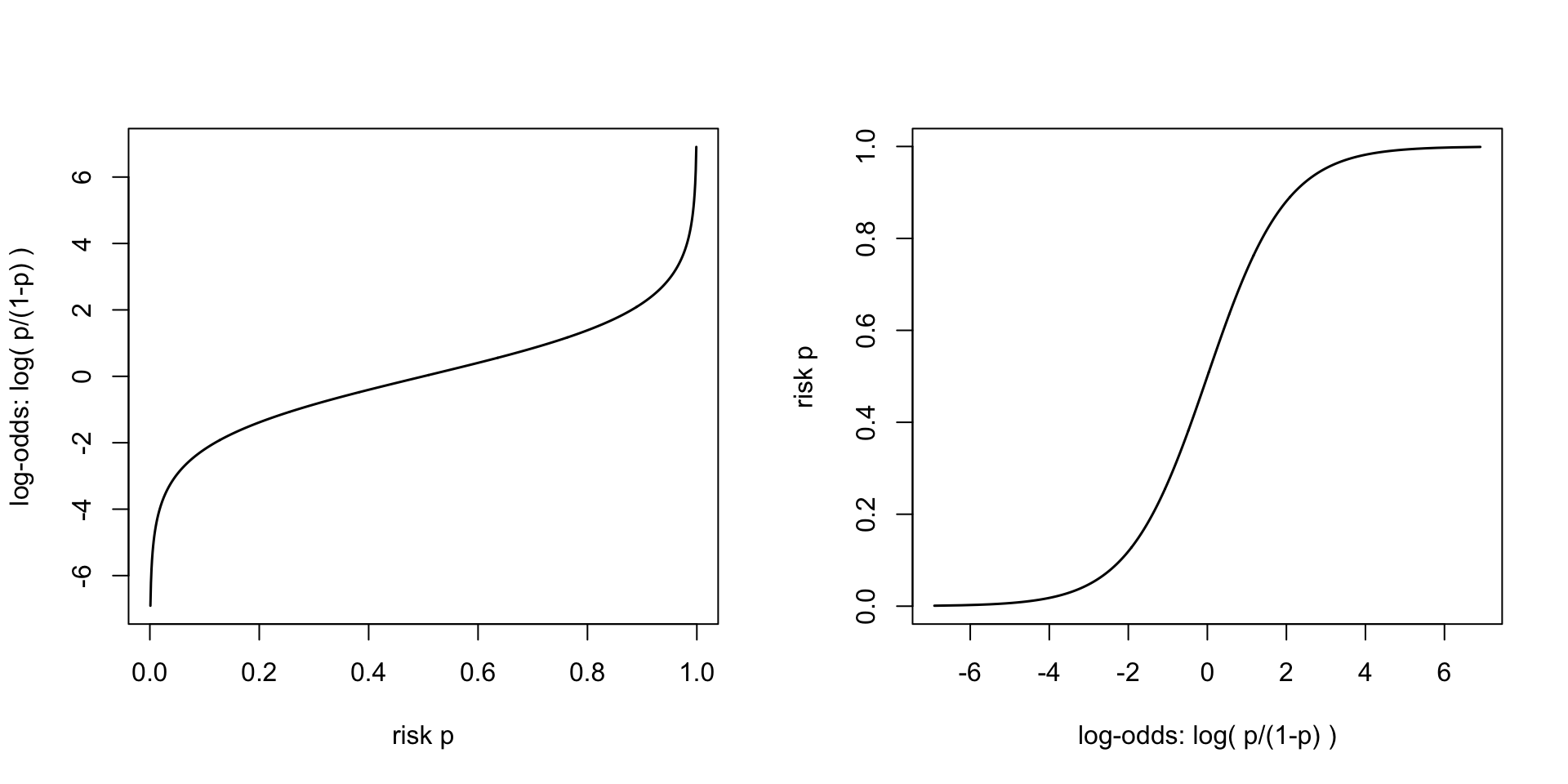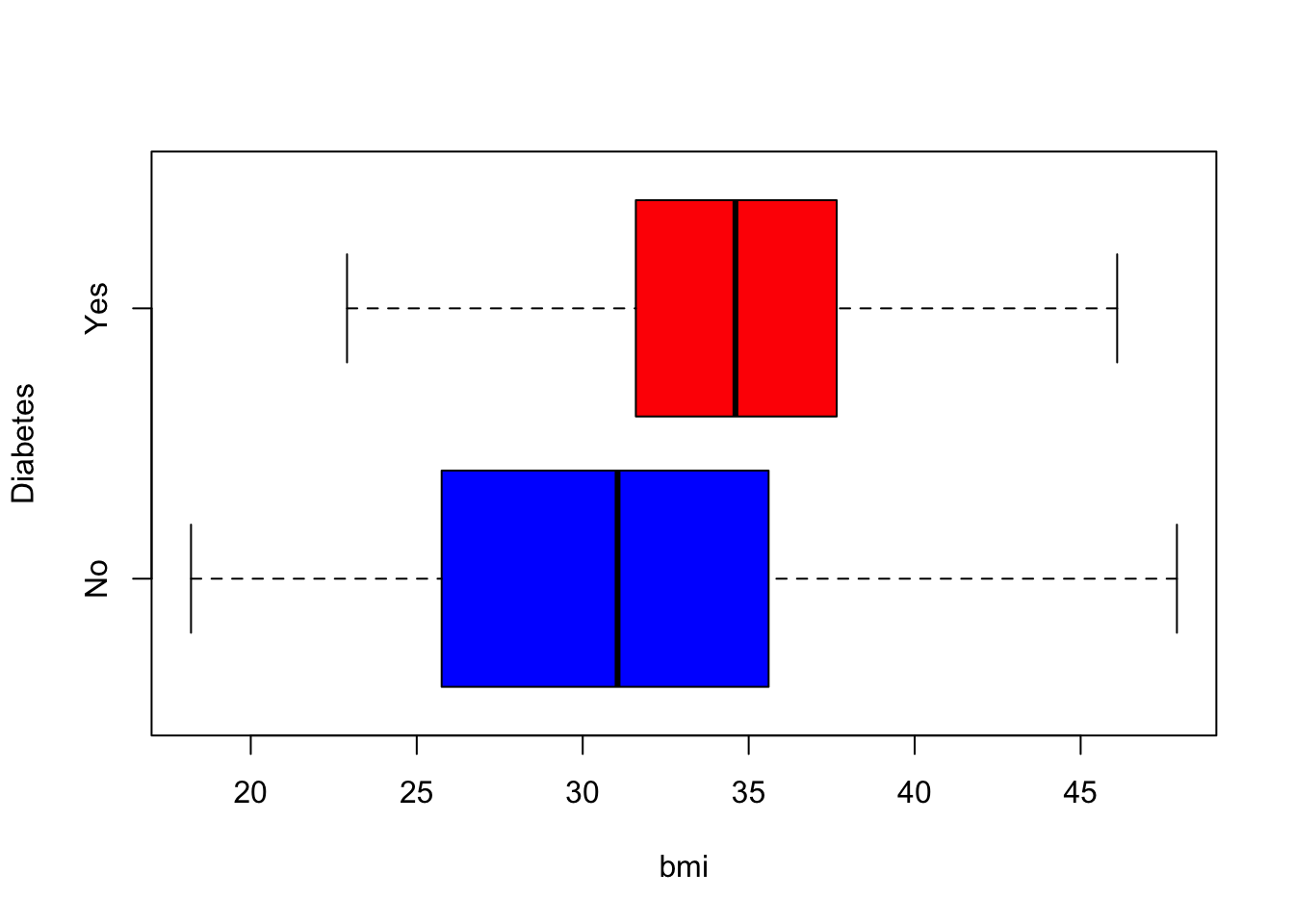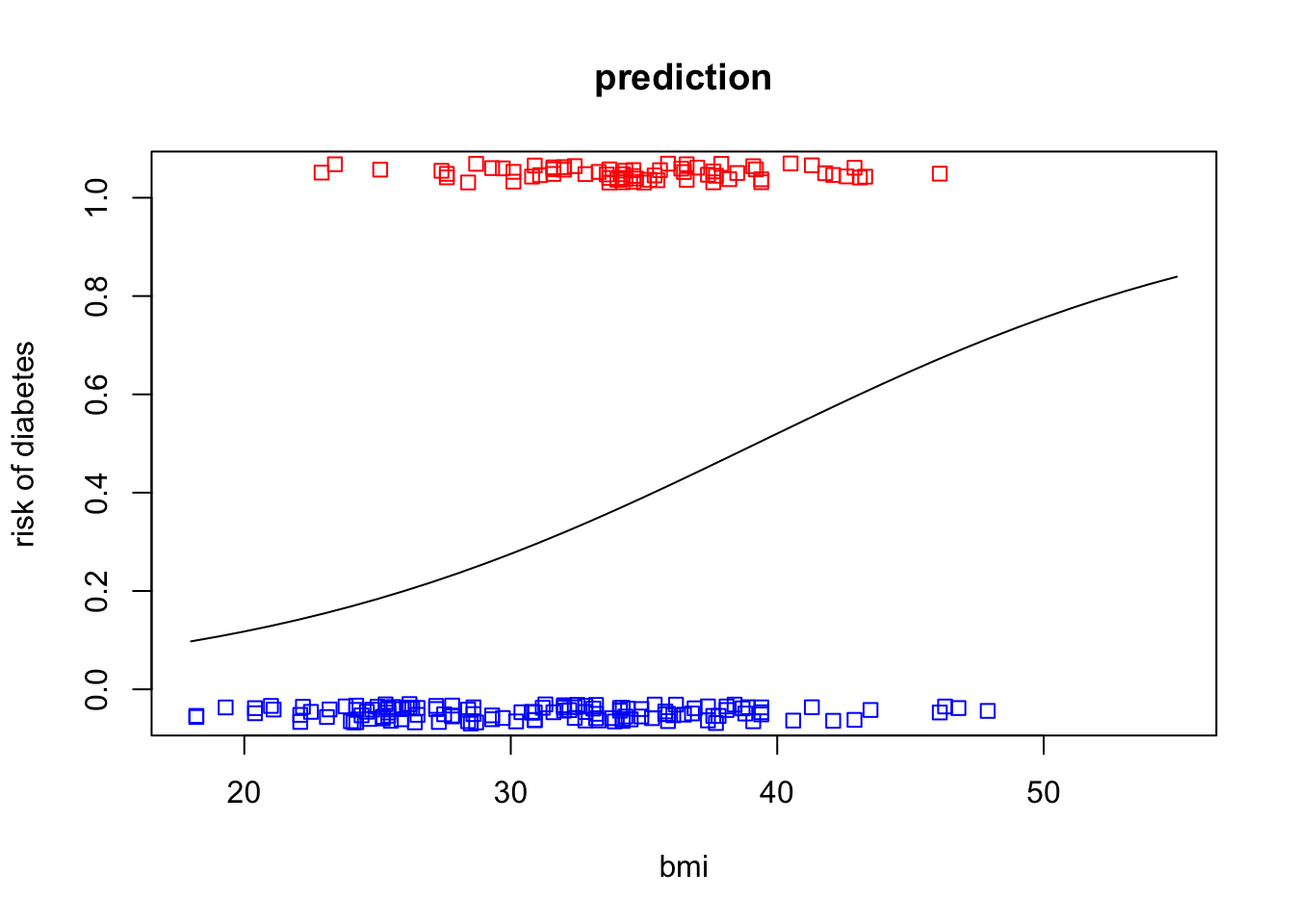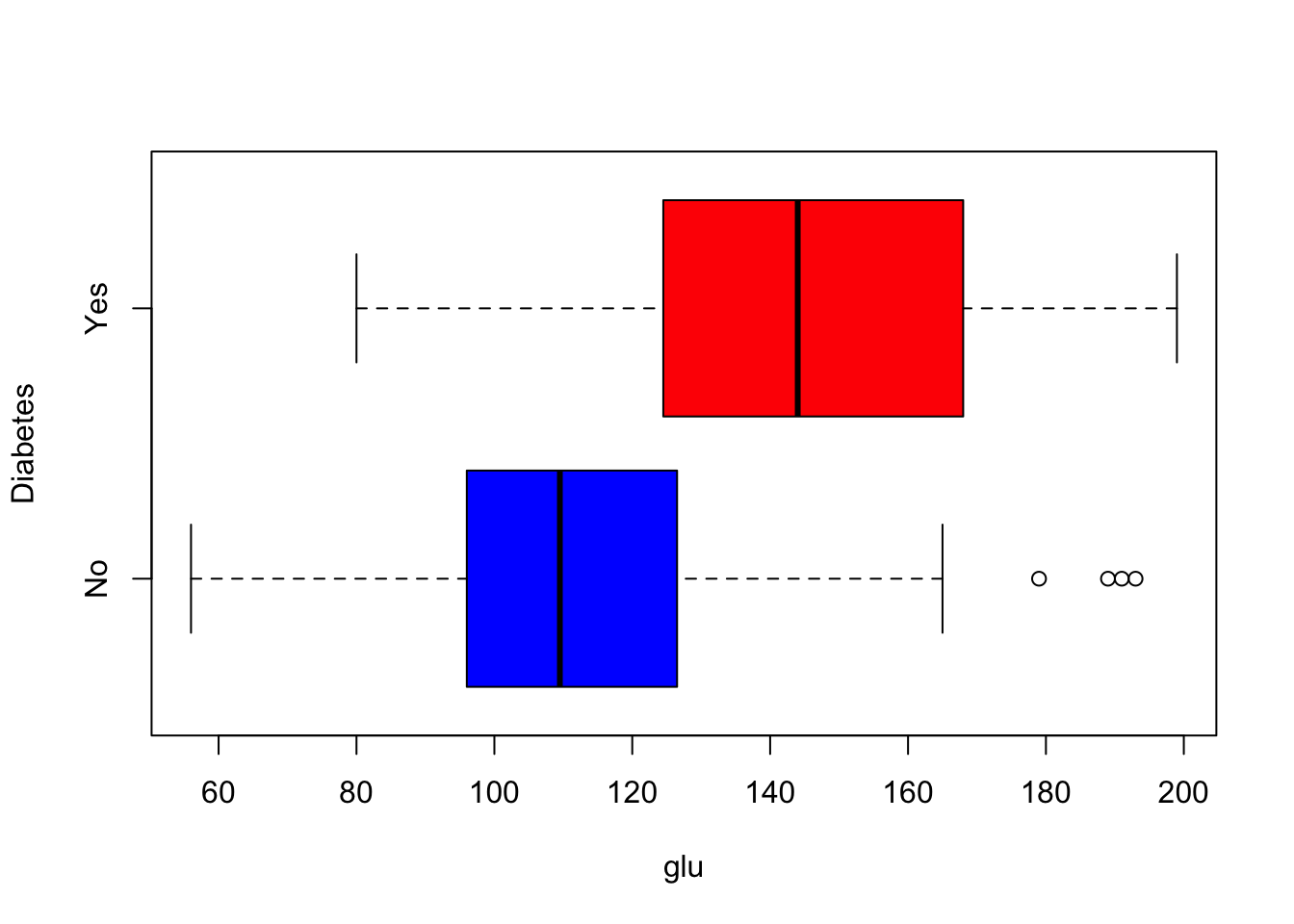In this lecture, we study a relationship between a binary variable, such as a disease status, and one or more predictors, such as risk factors of a disease. We extend the linear regression model to binary outcomes by a modeling approach called logistic regression.

### Modeling risk for binary outcomes through log-odds

When the outcome $$Y$$ of an experiment/observational study is binary (can take only two values, denoted by 0 and 1), we typically want to model how the probability $$p_i$$, that the outcome is 1 for individual $$i$$, depends on the available knowledge of the values of predictor variables $$X_1,\ldots,X_k$$ for individual $$i$$. We call this probability “risk” although in general it can be any probability, not necessarily related to a disease risk.

Intuitively, extending our experience from the linear regression model, we would like to have a model for risk like $p_i = a + b\, x_i + \varepsilon_i,$ from which we could estimate how a change in the predictor value $$x_i$$ changes the risk $$p_i$$. This has two problems. First, we can’t measure $$p_i$$ but we only observe binary outcome $$y_i$$ which is either 0 or 1 (e.g. for the patients we set $$y_i=1$$ and for the healthy we set $$y_i=0$$). Second, the risk value $$p_i$$ is a probability and hence always between 0 and 1, whereas the linear model above doesn’t impose such a restriction to the right hand side of the equation, which could result in inacceptable risk values from the model.

In this lecture, we leave the first problem for R to handle. The second problem is solved by transforming the outcome value $$p_i$$ from the interval (0,1) to the whole real line by the log-odds transformation, where $$p_i$$ is replaced by $\log\left(\frac{p_i}{1-p_i}\right).$

par(mfrow = c(1,2))
p = seq(0.001, 0.999, 0.001)
y = log( p/(1-p) )
plot(p, y, xlab = "risk p", ylab = "log-odds: log( p/(1-p) )", t = "l", lwd = 1.5)
plot(y, p, xlab = "log-odds: log( p/(1-p) )", ylab = "risk p", t = "l", lwd = 1.5)When $$p_i$$ is the probability that an event of interest happens, then $$1-p_i$$ is the probability that the event does not happen. The odds of the event are $$p_i / (1-p_i)$$ and they tell how many times more likely the event is to happen than not to happen. For example, if risk is 50%, then the odds are 1:1 = 1 and if risk is 2/3 then the odds are 2:1 = 2. Large odds (and also large log-odds) correspond to very probable events and small odds (and also small log-odds) correspond to very unlikely events. Mathematically, the inverse of the log-odds transformation $\ell = \log\left( \frac{p}{1-p}\right) \qquad \textrm{ is } \qquad p = \frac{\exp(\ell)}{1+\exp(\ell)}.$

By taking the logarithm of odds, we have a quantity that can take any real number as its value. Hence, it is conceptually valid to use a regression model where $\log\left(\frac{p_i}{1-p_i}\right) = a + b\,x_i.$ If $$b$$ in this model is positive, then, as $$x$$ increases, also the odds of the event increases and therefore also the probability of the event increases. If $$b$$ is negative, then as $$x$$ increases, also the odds of the event and hence also the probability of the event decreases. With the logistic regression model, for each pair of estimates of $$a$$ and $$b$$, we can do a prediction of risk $$p_i$$ for individual $$i$$ given his/her value for predictor $$x_i$$.

#### Example 8.1

1. Risk of a disease is 10%. What are the odds of the disease? And log-odds of the disease? How does odds and log-odds change when risk changes from 10% to (100% - 10%) = 90%?

Odds are $$p/(1-p) = 0.1/(1-0.1) = 0.1/0.9 = 1/9 \approx 0.111$$. Log-odds are $$\log(1/9) = -2.197.$$ If risk changes to 90%, then odds become $$9/1 = 9$$ and log-odds become $$\log(9) = -\log(1/9) = 2.197$$. Thus odds change to their inverse value, and log-odds changes the sign.

1. Suppose that log-odds of a disease follows a linear model $$a + b\, x$$, where $$x$$ is measured BMI and parameter values are $$a=-1$$ and $$b=0.01$$. Is increasing BMI a risk or protective factor for the disease? What is the risk of disease for an individual with bmi = 30? What about bmi = 40?

Since larger log-odds mean larger odds mean larger risk, a positive value of $$b$$ means that increasing BMI increases the risk.

a = -1
b = 0.01
x = c(30, 40)
logodds = a + b*x
p = exp(logodds)/(1 + exp(logodds))
data.frame(bmi = x, risk = p)
##   bmi      risk
## 1  30 0.3318122
## 2  40 0.3543437

## Logistic regression in R

Let’s use a dataset Pima.tr on 200 women from Pima population (a Native American population) from MASS package.

library(MASS)
y = Pima.tr
str(y)
## 'data.frame':    200 obs. of  8 variables:
##  $npreg: int 5 7 5 0 0 5 3 1 3 2 ... ##$ glu  : int  86 195 77 165 107 97 83 193 142 128 ...
##  $bp : int 68 70 82 76 60 76 58 50 80 78 ... ##$ skin : int  28 33 41 43 25 27 31 16 15 37 ...
##  $bmi : num 30.2 25.1 35.8 47.9 26.4 35.6 34.3 25.9 32.4 43.3 ... ##$ ped  : num  0.364 0.163 0.156 0.259 0.133 ...
##  $age : int 24 55 35 26 23 52 25 24 63 31 ... ##$ type : Factor w/ 2 levels "No","Yes": 1 2 1 1 1 2 1 1 1 2 ...

This data frame contains the following columns:

Variable Explanation
npreg number of pregnancies
glu plasma glucose concentration in an oral glucose tolerance test
bp diastolic blood pressure (mm Hg)
skin triceps skin fold thickness (mm)
bmi body mass index
ped diabetes pedigree function
age age in years
type Yes or No, for diabetic according to WHO criteria

Our outcome variable is the binary diabetes status type.

table(y$type) ## ## No Yes ## 132 68 Let’s start by seeing how bmi associates to type using a boxplot and a t-test. Since type is a factor (see above output from str()), we can make boxplots and the t-test of bmi with respect to the two levels of the factor by using the notation bmi ~ type. boxplot(bmi ~ type, data = y, xlab = "bmi", ylab = "Diabetes", horizontal = TRUE, col = c("blue", "red")) #turns boxplot horizontalt.test(bmi ~ type, data = y) ## ## Welch Two Sample t-test ## ## data: bmi by type ## t = -4.512, df = 171.46, p-value = 1.188e-05 ## alternative hypothesis: true difference in means between group No and group Yes is not equal to 0 ## 95 percent confidence interval: ## -5.224615 -2.044547 ## sample estimates: ## mean in group No mean in group Yes ## 31.07424 34.70882 There seems to be a difference of about 3.5 kg/m^2, 95%CI (2.0..5.2) between the groups. If we are given a value of BMI, what would be a risk of diabetes (in these data)? Let’s use logistic regression. We use glm( ) function that fits “generalized linear models”, and we specify logistic regression by parameter family = "binomial". This means that the outcome variable in regression is modeled as having a binomial distribution, where the success probability may depend on the values of predictors. For the model formula, the syntax is similar to the linear model lm(). Important: If you forget to specify family = "binomial" in glm( ), then the function fits a linear model, not a logistic model! glm.1 = glm(type ~ bmi, data = y, family = "binomial") summary(glm.1) #Always check from summary that you have fitted the correct model ## ## Call: ## glm(formula = type ~ bmi, family = "binomial", data = y) ## ## Coefficients: ## Estimate Std. Error z value Pr(>|z|) ## (Intercept) -4.11156 0.92806 -4.430 9.41e-06 *** ## bmi 0.10482 0.02738 3.829 0.000129 *** ## --- ## Signif. codes: 0 '***' 0.001 '**' 0.01 '*' 0.05 '.' 0.1 ' ' 1 ## ## (Dispersion parameter for binomial family taken to be 1) ## ## Null deviance: 256.41 on 199 degrees of freedom ## Residual deviance: 239.97 on 198 degrees of freedom ## AIC: 243.97 ## ## Number of Fisher Scoring iterations: 4 Coefficient for bmi is the increase in log-odds of diabetes per one unit increase in bmi and shows a clear statistical association with P ~ 1e-4. Let’s demonstrate what this means in terms of the risk of diabetes by applying predict( ) function for a sequence of bmi values. The default output from predict( ) on logistic regression object is in log-odds but we can ask the prediction directly as probabilities by specifying type = "response". bmi.grid = 18:55 data.in = data.frame(bmi = bmi.grid) y.pred = predict(glm.1, newdata = data.in, type = "response") #type = "response" predicts probabilities Let’s plot these predictions as a function of bmi, and let’s add to the figure also the bmi distributions of the observed cases and controls of diabetes using stripchart(). plot(bmi.grid, y.pred, ylim = c(-0.05,1.05), t = "l", main = "prediction", ylab = "risk of diabetes", xlab = "bmi") stripchart(y$bmi[y$type == "No"], at = -0.05, add = T, method = "jitter", jitter = 0.02, col = "blue") stripchart(y$bmi[y$type == "Yes"], at = 1.05, add = T, method = "jitter", jitter = 0.02, col = "red")We drew the predictions up to bmi = 55, but we see that original data included few observations above 45, and hence we shouldn’t expect the model to be reliable after that. We see that the risk raises from about 10% at bmi < 20 up to 60% at bmi > 40. While the relationship in this BMI range is close to linear, we still see that there is some non-linearity in the prediction curve, which is a difference from the corresponding linear regression model. Is this a good model? Let’s define some measures for how well the model explains the data. We use misclassification error and ROC curve for this purpose. #### Missclassification error We can predict the class (0 or 1) of each sample based on the model, for example, by predicting the class to be 1 if the predicted risk > 0.5 and otherwise predicting the class to be 0, and then see which proportion of the predictions are wrong. This proportion of wrong predictions is called the missclassification rate. Let’s apply it to our current data. We can use predict(glm.1, type = "response") to produce risks and then threshold them into 0 or 1 according to whether the risk is < 0.5 or > 0.5. pred.1 = predict(glm.1, type = "response") #if newdata is not specified, then predictions are given for original data y.pred.1 = ifelse(pred.1 < 0.5, 0, 1) #if pred.1[i] < 0.5 then sets y.pred.1[i]=0 else sets y.pred.1[i]=1 table(y.pred.1, y$type) #cross-tabulate predictions vs. true type
##
## y.pred.1  No Yes
##        0 120  57
##        1  12  11

We see that missclassification error is $$\frac{57+12}{200} = 0.345$$. Two measures often used in medical research are

• sensitivity, the proportion of true 1s that are correctly labeled as 1s: $$\frac{11}{57+11} = 0.162$$ and
• specificity, the proportion of true 0s that are correctly labeled as 0s: $$\frac{120}{120+12} = 0.909$$.

The classifier is perfect if and only if it has both sensitivity and specificity equal to 1. Here our model has a very low sensitivity of 16% and a higher specificity of 91%. Low sensitivity means that the model doesn’t really detect well the true diabetes cases.

Let’s add glu to the model. It is expected to predict strongly diabetes, since it is used to diagnose diabetes.

boxplot(glu ~ type, data = y, xlab = "glu", ylab = "Diabetes", horizontal = T, col = c("blue","red"))t.test(glu ~ type, data = y)
##
##  Welch Two Sample t-test
##
## data:  glu by type
## t = -7.3856, df = 121.76, p-value = 2.081e-11
## alternative hypothesis: true difference in means between group No and group Yes is not equal to 0
## 95 percent confidence interval:
##  -40.51739 -23.38813
## sample estimates:
##  mean in group No mean in group Yes
##          113.1061          145.0588
glm.2 = glm(type ~ bmi + glu, data = y, family = "binomial")
summary(glm.2)
##
## Call:
## glm(formula = type ~ bmi + glu, family = "binomial", data = y)
##
## Coefficients:
##              Estimate Std. Error z value Pr(>|z|)
## (Intercept) -8.216106   1.346965  -6.100 1.06e-09 ***
## bmi          0.090016   0.031268   2.879  0.00399 **
## glu          0.035716   0.006311   5.659 1.52e-08 ***
## ---
## Signif. codes:  0 '***' 0.001 '**' 0.01 '*' 0.05 '.' 0.1 ' ' 1
##
## (Dispersion parameter for binomial family taken to be 1)
##
##     Null deviance: 256.41  on 199  degrees of freedom
## Residual deviance: 198.47  on 197  degrees of freedom
## AIC: 204.47
##
## Number of Fisher Scoring iterations: 4

Let’s see the missclassification rate in the updated model.

pred.2 = predict(glm.2, type = "response")
y.pred.2 = ifelse(pred.2 < 0.5, 0, 1)
table(y.pred.2, y$type) #cross-tabulate predictions vs. true type ## ## y.pred.2 No Yes ## 0 116 31 ## 1 16 37 Missclassification rate is now $$\frac{31+16}{200} = 0.235$$ and has clearly fallen from its earlier value of 0.345 when BMI was alone in the model. Missclassification rate is an intuitive measure, but it has a downside that it is defined by a particular fixed risk threshold (usually 0.5). We can get more information about a classifier, if we can see its performance throughout all possible risk cutoffs. The ROC curve gives us such information. #### ROC curve In receiver operating characteristics (ROC) curve, we run through all possible risk thresholds to classify samples into 1s and 0s and, for each threshold, we compute the true positive rate (= sensitivity, the proportion of true 1s that are labeled as 1s) against false positive rate (=1-specificity, the proportion of true 0s that are labeled as 1s). Let’s draw ROC curves from models 1 and 2 above, and discuss the curves. p.thresh = seq(-0.001, 1.001, 0.001) #risk thresholds for which ROC is computed #We make roc.1 and roc.2 matrices here and then fill them in the for-loop below roc.1 = matrix(NA, ncol = 2, nrow = length(p.thresh)) #will have col1 = FP rate and col2 = TP rate roc.2 = matrix(NA, ncol = 2, nrow = length(p.thresh)) for(ii in 1:length(p.thresh)){ #goes through all risk thresholds p = p.thresh[ii] # now p is the current risk threshold y.pred.1 = ifelse(pred.1 < p, 0, 1) #0-1 predictions based on current risk threshold 'p' y.pred.2 = ifelse(pred.2 < p, 0, 1) roc.1[ii,1] = sum( y$type == "No" & y.pred.1 == 1) / sum( y$type == "No" ) #false positive rate roc.1[ii,2] = sum( y$type == "Yes" & y.pred.1 == 1) / sum( y$type == "Yes") #true positive rate roc.2[ii,1] = sum( y$type == "No" & y.pred.2 == 1) / sum( y$type == "No" ) roc.2[ii,2] = sum( y$type == "Yes" & y.pred.2 == 1) / sum( y\$type == "Yes")
}

plot(NULL, xlim = c(0,1), ylim = c(0,1), main = "ROC",
xlab = "False positive rate", ylab = "True positive rate") #Makes an empty plot
lines(roc.1, col = "skyblue", lwd=1.5) #adds ROC of model 1
lines(roc.2, col = "darkblue", lwd=1.5) #adds ROC of model 2

legend("bottomright", leg = c("bmi", "bmi+glu"), col = c("skyblue", "darkblue"), lwd = 1.5)
abline(a = 0, b = 1, lty = 2) #adds diagonal line Y = X